Next: Time evolution of eccentricity Up: Perihelion precession of Mercury Previous: Perihelion precession of Mercury

# Introduction

As described in Section 5.5, the ability of general relativity to explain the discrepancy between the observed perihelion precession rate of Mercury, and that calculated from Newtonian mechanics, was one of the first major successful tests of this theory. However, given that the Newtonian precession rate is about ten times larger than the general relativistic correction, it is necessary to calculate the Newtonian rate to great accuracy in order for this particular test to be convincing. In this Appendix, we perform such a calculation, adapting the method outlined by Stewart (Stewart 2005).

Let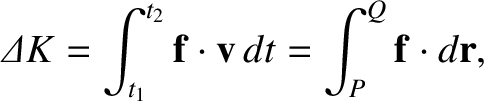and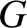be the position vectors of Mercury and some other planet in the solar system, respectively, relative to the Sun. Furthermore, let,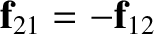, and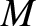be the masses of Mercury, the other planet, and the Sun, respectively. According to the analysis of Section 10.2, the vectorevolves in time according to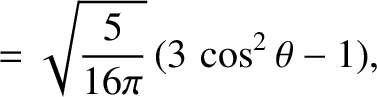(B.1)

where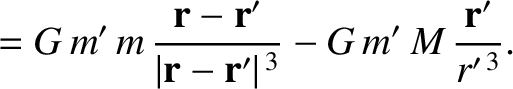. Here, the disturbing function due to the other planet takes the form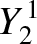(B.2)

where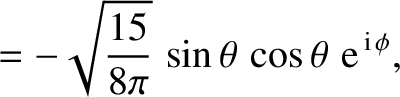.Next: Time evolution of eccentricity Up: Perihelion precession of Mercury Previous: Perihelion precession of Mercury
Richard Fitzpatrick 2016-03-31